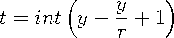Functions and CALL Routines

# DEPDBSL Function

Returns the declining balance with conversion to a straight-line depreciation.
 Category: Financial

## Syntax

 DEPDBSL(p,v,y,r)

### Arguments

p

is an integer, the period for which the calculation is to be done.

v

is numeric, the depreciable initial value of the asset.

y

is an integer, the lifetime of the asset.

 Range: y > 0
r

is numeric, the rate of depreciation that is expressed as a fraction.

 Range: r0

The DEPDBSL function returns the depreciation by using the declining balance method with conversion to a straight-line depreciation, which is given by the following equation:whereand int( ) denotes the integer part of a numeric argument.

The p and y arguments must be expressed by using the same units of time. The declining balance that changes to a straight-line depreciation chooses for each time period the method of depreciation (declining balance or straight-line on the remaining balance) that gives the larger depreciation.

An asset has a depreciable initial value of \$1,000 and a ten-year lifetime. Using a declining balance rate of 150 percent, the depreciation of the value of the asset in the fifth year can be expressed as

`   y5=depdbsl(5,1000,10,1.5);`

The value 87.001041667 is returned. The first and the third arguments are expressed in years.Previous Page | Next Page | Top of Page